# Coevolving the "Ideal" Trainer

## Description of the Problem

#### One-dimensional Cellular Automata

A one-dimensional cellular automaton (CA) is a linear wrap-around array composed of N cells in which each cell can take one out of k possible states. A rule is defined for each cell in order to update its state. This rule determines the next state of a cell given its current state and the state of cells in a predefined neighborhood. For the model discussed in this proposal, this neighborhood is composed of cells whose distance is at most r from the central cell. This operation is performed synchronously for all the cells in the CA. For the work presented in this proposal, the state of cells is binary (k = 2), N = 149 and r = 3. This means that the size of the rule space is 2^{2^{2 * r + 1}} = 2^{128}.

Cellular automata have been studied widely as they represent one of the simplest systems in which complex emergent behaviors can be observed. This model is very attractive as a means to study complex systems in nature. Indeed, the evolution of such systems is ruled by simple, locally-interacting components which result in the emergence of global, coordinated activity.

#### The Majority Function

The task consists in discovering a rule for the one-dimensional CA which implements the majority function as accurately as possible. This is a density classification task, for which one wants the state of the cells of the CA to relax to all 0's or 1's depending on the density of the initial configuration (IC) of the CA, within a maximum of M time steps. Following [Mitchell et al., 1994], $\rho_c$ denotes the threshold for the classification task (here, $\rho_c = 1/2$), $\rho$ denotes the density of 1's in a configuration and $\rho_0$ denotes the density of 1's in the initial configuration. Figure 1 presents two examples of the space-time evolution of a CA with $\rho_0 < \rho_c$ on the left and $\rho_0 > \rho_c$ on the right. The initial configuration is at the top of the diagram and the evolution in time of the different configurations is represented downward.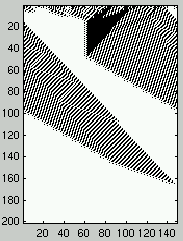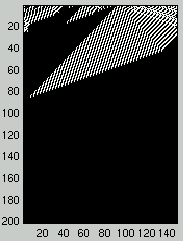Figure 1: Two space-time diagrams describing the evolution of CA states.

The task $\rho_c = 1/2$ is known to be difficult. In particular, it has been proven that no rule exists that will result in the CA relaxing to the correct state for all possible ICs [Land & Belew, 1995]. Indeed, the density is a global property of the initial configuration while individual cells of the CA have access to local information only. Discovering a rule that will display the appropriate computation by the CA with the highest accuracy is a challenge, and the upper limit for this accuracy is still unknown. Table 1 describes the performance for that task for different published rules and different values of N along with a new best rule discovered using the coevolutionary approach presented in this document.

table 1: Performance of different published CA rules and the new best rule discovered by coevolution for the majority function.

 N 149 599 999 Coevolution 0.863 +/- 0.001 0.822 +/- 0.001 0.804 +/- 0.001 Das rule 0.823 +/- 0.001 0.778 +/- 0.001 0.764 +/- 0.001 ABK rule 0.824 +/- 0.001 0.764 +/- 0.001 0.730 +/- 0.001 GKL rule 0.815 +/- 0.001 0.773 +/- 0.001 0.759 +/- 0.001

The Gacs-Kurdyumov-Levin (GKL) rule was designed in 1978 for a different goal than the $\rho_c = 1/2$ task [Mitchell et al., 1994]. However, for a while it provided the best known performance. [Mitchell et al., 1994] and [Das et al., 1994] used Genetic Algorithms (GAs) to explore the space of rules. This work resulted in an analysis of some of the complex behaviors exhibited by CAs using particles''. The GKL and Das rules are human-written while the Andre-Bennett-Koza (ABK) rule has been discovered using the Genetic Programming paradigm [Andre et al., 1996].

## Learning in a Fixed Environment: Evolution

• #### Canonical model of evolution

The traditional model for evolutionary approaches to problem solving is to design a representation for solutions and a fitness function. The absolute performance of individuals is evaluated with respect to that fitness function and search operators are used to explore the state space.

As an example, we implemented such an evolutionary approach for the discovery of CA rules for the $\rho_c = 1/2$ task. This implementation is similar to the one described in [Mitchell et al., 1994]. Each rule is coded on a binary string of length $2^{2 * r + 1} = 128$. One-point crossover is used with a 2%$bit mutation probability. The population size is n_R = 200 for rules and n_{IC} = 200 for ICs. The population of ICs is composed of binary strings of length N = 149. This population is fixed and it is initialized according to a uniform distribution over$\rho_0 \in [0.0, 1.0]$. The population of rules is also initialized according to a uniform distribution over$[0.0, 1.0]$for the density. A natural definition for the fitness of rules is the number of Initial Configurations (ICs) for which the CA relaxes to the correct state: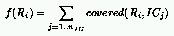where:Figure 2 describes the evolution of the distribution of the density of rules over time. A common behavior resulting from such an evolutionary setup is that the entire population focuses quickly to a small domain of the search space in the neighborhood of the current best individual. Eventually, some progress is observed over time. The variance for the final performance over several runs is usually large.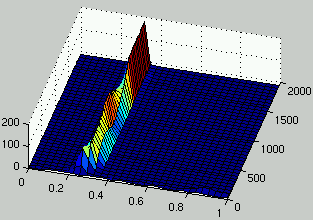Figure 2: Evolution of the distribution of the density of rules over time. • #### Maintaining diversity by introducing resource sharing Several techniques have been designed to improve search in a fixed landscape. Usually those techniques maintain diversity in the population in order to avoid premature convergence. [Mahfoud, 1995] presents different niching techniques that are useful in particular for the exploration of multi-modal landscape. A technique that we successfully exploited in the past to maintain diversity is called resource sharing [Juillé & Pollack, 1996]. Resource sharing implements a coverage-based heuristic by giving a higher payoff to problems that few individuals can solve. One way to implement this technique for the evolution of CA rules is to define the fitness of rules as follows: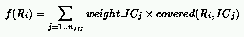where: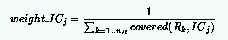In this definition, the weight of an IC corresponds to the payoff it returns if a rule covers it. If few rules cover an IC, this weight will be much larger than if a lot of rules cover that same IC. Figure 3 shows the evolution of the distribution of rules density over several generations. It can be observed that diversity is maintained for about 500 generations during which different alternatives are explored simultaneously by the population of rules. Then, a solution is discovered that moves the search in the area where density is close to 0.5. Afterward, several niches might still be explored, however the coding used to construct the figures doesn't allow the representation of those different niches since all the rules have a similar density. Usually, this technique results in better performance on average. However, it takes also more time to converge. This is a trade-off between speed and quality of solutions.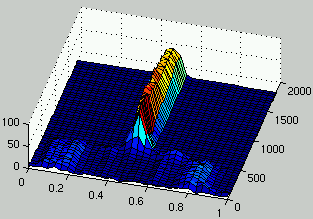Figure 3: Evolution of the distribution of the density of rules over time. ## Learning in an Adapting Environment: Coevolution The idea of using coevolution in search was introduced by [Hillis, 1992]. In his work, a population of parasites coevolves with a population of sorters. The goal of parasites is to exploit weaknesses of sorters by discovering input vectors that sorters cannot solve correctly while sorters evolve strategies to become perfect sorting networks. In coevolution, individuals are evaluated with respect to other individuals instead of a fixed environment (or landscape). As a result, agents adapt in response to other agents' behavior. For the work presented in this document, I will define coevolutionary learning as a learning procedure involving the coevolution of two populations: a population of learners and a population of problems. Moreover, the fitness of an individual in a population is defined only with respect to members of the other population. Two cases can be considered in such a framework, depending on whether the two populations benefit from each other or whether they have different interests (i.e., if they are in conflict). Those two modes of interaction are called cooperative and competitive respectively. In the following paragraphs, those modes of interaction are described experimentally in order to stress the different issues related to coevolutionary learning. • #### Cooperation between populations In this mode of interaction, improvement on one side results in positive feedback on the other side. As a result, there is a reinforcement of the relationship between the two populations. From a search point of view, this can be seen as an exploitative strategy. Agents are not encouraged to explore new areas of the search space but only to perform local search in order to further improve the strength of the relationship. Following a natural extension of the evolutionary case to the cooperative model, the fitness of rules and ICs can be defined as follows for the$\rho_c = 1/2$task:For our experiments, the same setup as the one described previously is used. The population size is n_R = 200 for rules and n_{IC} = 200 for ICs. The population of rules and ICs are initialized according to a uniform distribution over$[0.0, 1.0]$for the density. At each generation, the top 95% of each population reproduces to the next generation and the remaining 5% is the result of crossover between parents from the top 95% selected using a fitness proportionate rule. Figure 4 presents the evolution of the density of rules and ICs for one run using this cooperative model. Without any surprise, the population of rules and ICs quickly converge to a domain of the search space where ICs are easy for rules and rules consistently solve ICs. There is little exploration of the search space.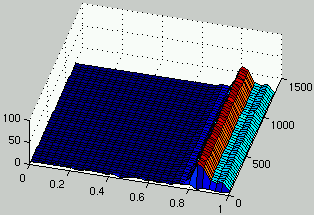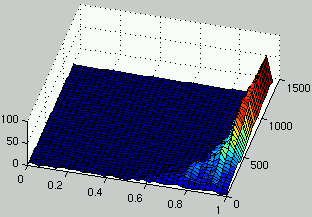Figure 4: Evolution of the distribution of the density of rules (left) and the density of ICs (right) over time. • #### Competition between populations In this mode of interaction, the two populations are in conflict. Improvement on one side results in negative feedback for the other population. Two outcomes are possible: • Unstable behavior: alternatively, agents in one population outperform members of the other population until those discover a strategy to defeat the first one and vice versa. This results in an unstable behavior where species appear then disappears and reappears again in a later generation. • a Stable state is reached: this happens if one population consistently outperforms the other. For instance, a very strong strategy is discovered by agents in one population. For the$\rho_c = 1/2$task, the fitness defined in the cooperative case can be modified as follows to implement the competitive model: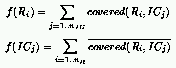Here, the goal of the population of rules is to discover rules that defeat ICs in the other population by allowing the CA to relax to the correct state, while the goal of ICs is to defeat the population of rules by discovering initial configurations that are difficult to classify. Figure 5 describes an example of a run using this definition of the fitness. In a first stage, the two populations exhibit a cyclic behavior. Rules with low density have an advantage because there are more ICs with low density. In response to this, ICs with high density have an evolutionary advantage and have more representatives in the population. In turn, rules with high density get an evolutionary advantage\ldots This unstable behavior exhibited by the two populations is an instance of the Red Queen effect [Cliff & Miller, 1995]: fitness landscapes are changing dynamically as a result of agents from each population adapting in response to the evolution of members of the other population. The performance of individuals is evaluated in a changing environment, making continuous progress difficult. A typical consequence is that agents have to learn again what they already knew in the past. In the context of evolutionary search, this means that domains of the state space that have already been explored in the past are searched again. Then, a stable state is reached: in this case, the population of rules adapts faster than the population of ICs, resulting in a population focusing only on rules with high density and eliminating all instances of low density rules (a finite population is considered). Then, low density ICs exploit those rules and overcome the entire population.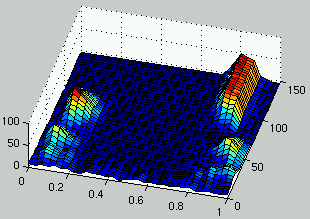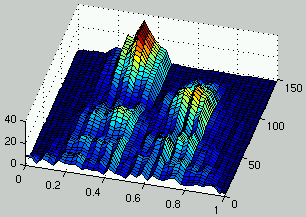Figure 5: Evolution of the distribution of the density of rules (left) and the density of ICs (right) over time. • #### Resource sharing and mediocre meta-stable states We have seen that by introducing resource sharing, it is possible to explore simultaneously different alternatives by maintaining several niches in the population. This section presents some experiments where resource sharing is introduced in the competitive mode of interaction presented in the previous section. The fitness of rules and ICs is then defined as follows: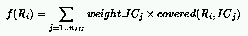where: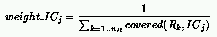and:where: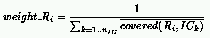This framework allows the presence of multiple niches in both populations, each niche corresponding to a particular competence relevant to defeat some members of the other population. Figure 6 describes one run for this definition of the interaction between the two populations. It can be seen that the unstable behavior which was observed in the previous section doesn't occur anymore and that two species coexist in the population of rules: a species for low density rules and another one for high density rules. Those two species drive the evolution of ICs towards the domain of initial configurations that are most difficult to classify (i.e.,$\rho_0 = 1/2$). However, the two populations have entered a mediocre meta-stable state. This means that multiple average performance niches coexist in both populations in a stable manner.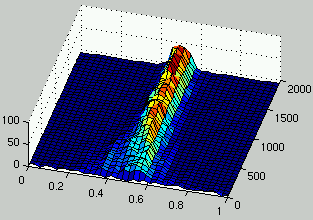Figure 6: Evolution of the distribution of the density of rules (left) and the density of ICs (right) over time. ## Coevolving the "Ideal" Trainer The central idea of the coevolutionary learning approach consists in exposing learners to problems that are just beyond those they know how to solve. By maintaining this constant pressure towards slightly more difficult problems, a arms race among learners is induced such that learners that adapt better have an evolutionary advantage. The underlying heuristic implemented by this arms race is that {\em adaptability} is the driving force for improvement. In order to achieve this result, our system tries to satisfy the following two goals: • to provide an optimum'' gradient for search. This means that the training environment defined by the population of problems can determine reliably which learners are the most promising at each stage of the search process. This means that problems must be informative. If problems are too difficult or too easy, learners get little feedback and there is no gradient to determine in which direction the search should focus. • to allow continuous progress. The goal is to avoid the Red Queen effect by providing a training environment which continues to test learners about problems they solved in the past. In a sense, the training environment must also play the role of memory. The difficulty resides in the accurate implementation of those concepts in a search algorithm. ### Experimental Results It is believed, that ICs become more and more difficult to classify correctly as their density gets closer to the$\rho_c$threshold. Therefore, our idea is to construct a framework that adapts the distribution of the density for the population of ICs as CA-rules are getting better to solve the task. The following definition for the fitness of rules and ICs has been used to achieve this goal: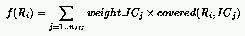where: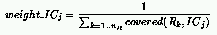and: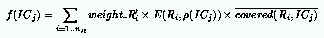where: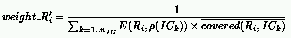This definition implements the competitive relationship with resource sharing. However, a new component, namely$E(R_i, \rho(IC_j))$, has been added in the definition of the ICs' fitness. The purpose of this new component is to give a low payoff to IC_j if ICs with density$\rho(IC_j)$return little information with respect to the rule R_i. We consider that information is collected if rule R_i does better or worse than random guessing over ICs with density$\rho(IC_j)$(that is, R_i covers strictly more or strictly less than 50% of those ICs). Following this idea, we defined E() as the complement of the entropy of the outcome between a rule and ICs with a given density: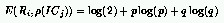where: p is the probability that an IC with density$\rho(IC_j)$defeats the rule R_i and q = 1 - p. Figures 7 and 8 describe the evolution of the density of rules and ICs for two runs. It can be seen that, as rules improve, the density of ICs is distributed on two peaks on each side of$\rho = 1/2\$. In the case of figure 7, it is only after 1,300 generations that a significant improvement is observed for rules. It is only at that time that the population of ICs adapts dramatically in order to propose more challenging initial configurations.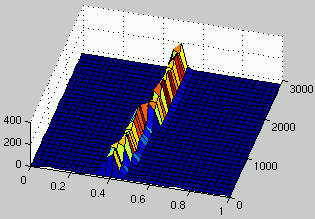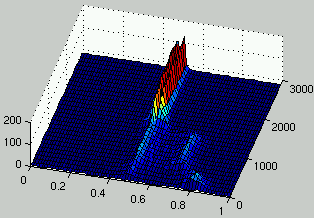Figure 7: Evolution of the distribution of the density of rules (left) and the density of ICs (right) over time.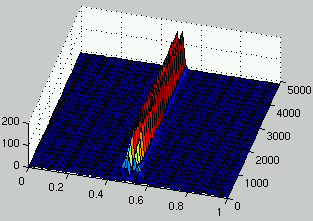Figure 8: Evolution of the distribution of the density of rules (left) and the density of ICs (right) over time.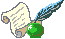Coevolutionary Learning: a Case Study (with J. B. Pollack), Proceedings of the Fifteenth International Conference on Machine Learning (ICML-98) , Madison, Wisconsin, USA, July 24-26, 1998, to appear.Coevolving the Ideal'' Trainer: Application to the Discovery of Cellular Automata Rules (with J. B. Pollack), Proceedings of the Third Annual Genetic Programming Conference (GP-98) , Madison, Wisconsin, USA, July 22-25, 1998, to appear.

### References

[Andre et al., 1996] Andre, D., Bennet, F. H. & Koza, J. R. (1996). Evolution of intricate long-distance communication signals in cellular automata using genetic programming. In Artificial Life V: Proceedings of the Fifth International Workshop on the Synthesis and Simulation of Living Systems. Nara, Japan.

[Cliff & Miller, 1995] Cliff, D. & Miller, G. F. (1995). Tracking the red queen: Measurements of adaptive progres in co-evolutionary simulations. In the Proceedings of the Third European Conference on Artificial Life, pp. 200-218. Springer-Verlag. LNCS 929.

[Das et al., 1994] Das, R., Mitchell, M. & Crutchfield, J. P. (1994). A genetic algorithm discovers particle-based computation in cellular automata. In Parallel Problem Solving from Nature - PPSN III, pp. 344-353. Springer-Verlag. LNCS 866.

[Hillis, 1992] Hillis, W. D. (1992). Co-evolving parasites improves simulated evolution as an optimization procedure. In Langton, C. et al. (Eds), Artificial Life II, pp. 313-324. Addison Wesley.

[Juillé & Pollack, 1996] Juillé, H. & Pollack, J.B. (1996). Co-evolving intertwined spirals. In Proceedings of the Fifth Annual Conference on Evolutionary Programming, pp. 461-468. MIT Press.

[Land & Belew, 1995] Land, M. & Belew, R. K. (1995). No perfect two-state cellular automata for density classification exists. Physical Review Letters, 74(25):1548-1550.

[Mahfoud, 1995] Mahfoud, S. W. (1995). Niching methods for genetic algorithms. Technical report, University of Illinois at Urbana-Champaign. IlliGAL Report No. 95001.

[Mitchell et al., 1994] Mitchell, M., Crutchfield, J.P. & Hraber, P.T. (1994). Evolving cellular automata to perform computations: Mechanisms and impediments. Physica D, 75:361-391.